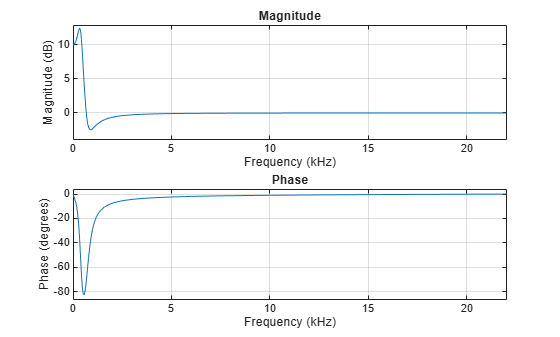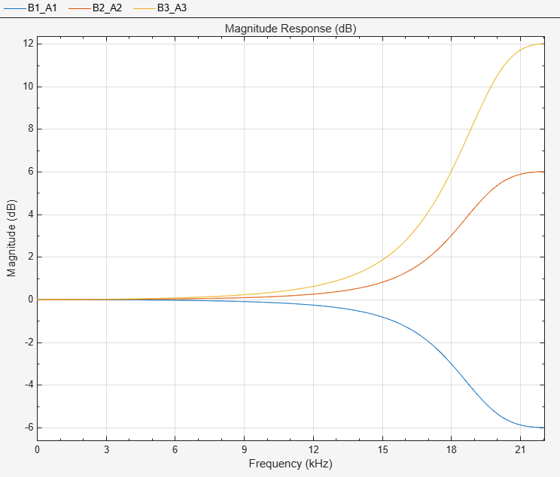Documentation

# designShelvingEQ

Design shelving equalizer

## Syntax

``````[B,A] = designShelvingEQ(gain,slope,Fc)``````
``````[B,A] = designShelvingEQ(gain,slope,Fc,type)``````
``````[B,A] = designShelvingEQ(___,Name,Value)``````

## Description

example

``````[B,A] = designShelvingEQ(gain,slope,Fc)``` designs a low-shelf equalizer with the specified gain, slope, and cutoff frequency, `Fc`. The equalizer is returned as cascaded second-order section (SOS) IIR filters.```

example

``````[B,A] = designShelvingEQ(gain,slope,Fc,type)``` specifies the design type as a low-shelving or high-shelving equalizer.```
``````[B,A] = designShelvingEQ(___,Name,Value)``` specifies options using one or more `Name,Value` pair arguments.```

## Examples

collapse all

Design three second-order IIR low-shelf equalizers using `designShelvingEQ`. The three shelving equalizers use three separate slope specifications.

Specify sampling frequency, peak gain, slope coefficient, and normalized cutoff frequency for three shelving equalizers. The sampling frequency is in Hz. The peak gain is in dB.

```Fs = 44.1e3; gain = 5; slope1 = 0.5; slope2 = 0.75; slope3 = 1; Fc = 1000/(Fs/2);```

Design the filter coefficients using the specified parameters.

```[B1,A1] = designShelvingEQ(gain,slope1,Fc,"Orientation","row"); [B2,A2] = designShelvingEQ(gain,slope2,Fc,"Orientation","row"); [B3,A3] = designShelvingEQ(gain,slope3,Fc,"Orientation","row");```

```fvtool( ... dsp.BiquadFilter([B1,A1]), ... dsp.BiquadFilter([B2,A2]), ... dsp.BiquadFilter([B3,A3]), ... "Fs",Fs, ... "FrequencyScale","Log"); legend("slope = 0.5", ... "slope = 0.75", ... "slope = 1");```Create audio file reader and audio device writer objects. Use the sample rate of the reader as the sample rate of the writer. Call `setup` to reduce the computational load of initialization in an audio stream loop.

```frameSize = 256; fileReader = dsp.AudioFileReader( ... "RockGuitar-16-44p1-stereo-72secs.wav", ... "SamplesPerFrame",frameSize); sampleRate = fileReader.SampleRate; deviceWriter = audioDeviceWriter( ... "SampleRate",sampleRate); setup(fileReader) setup(deviceWriter,ones(frameSize,2))```

Play the audio signal through your device.

```count = 0; while count < 2500 audio = step(fileReader); play(deviceWriter,audio); count = count + 1; end reset(fileReader)```

Design a second-order sections (SOS) low-shelf equalizer.

```gain = 10; slope = 3; Fc = 0.025; [B,A] = designShelvingEQ(gain,slope,Fc);```

```SOS = [B',[1,A']]; fvtool(dsp.BiquadFilter("SOSMatrix",SOS), ... "Fs",fileReader.SampleRate, ... "FrequencyScale","Log");``````myFilter = dsp.BiquadFilter( ... "SOSMatrixSource","Input port", ... "ScaleValuesInputPort",false);```

Create a spectrum analyzer object to visualize the original audio signal and the audio signal passed through your low-shelf equalizer.

```scope = dsp.SpectrumAnalyzer( ... "SampleRate",sampleRate, ... "PlotAsTwoSidedSpectrum",false, ... "FrequencyScale","Log", ... "FrequencyResolutionMethod","WindowLength", ... "WindowLength",frameSize, ... "Title","Original and Equalized Signal", ... "ShowLegend",true, ... "ChannelNames",{'Original Signal','Equalized Signal'});```

Play the equalized audio signal and visualize the original and equalized spectrums.

```setup(scope,ones(frameSize,2)) count = 0; while count < 2500 originalSignal = fileReader(); equalizedSignal = myFilter(originalSignal,B,A); scope([originalSignal(:,1),equalizedSignal(:,1)]); deviceWriter(equalizedSignal); count = count + 1; end```

As a best practice, release your objects once done.

```release(fileReader) release(deviceWriter) release(scope)```Design three second-order IIR high shelf equalizers using `designShelvingEQ`. The three shelving equalizers use three separate gain specifications.

Specify sampling frequency, peak gain, slope coefficient, and normalized cutoff frequency for three shelving equalizers. The sampling frequency is in Hz. The peak gain is in dB.

```Fs = 44.1e3; gain1 = -6; gain2 = 6; gain3 = 12; slope = 0.8; Fc = 18000/(Fs/2);```

Design the filter coefficients using the specified parameters.

```[B1,A1] = designShelvingEQ(gain1,slope,Fc,"hi","Orientation","row"); [B2,A2] = designShelvingEQ(gain2,slope,Fc,"hi","Orientation","row"); [B3,A3] = designShelvingEQ(gain3,slope,Fc,"hi","Orientation","row");```

```fvtool([B1,A1;[1 0 0 1 0 0]], ... [B2,A2;[1 0 0 1 0 0]], ... [B3,A3;[1 0 0 1 0 0]], ... "Fs",Fs); legend("gain = -6 dB", ... "gain = 6 dB", ... "gain = 12 dB", ... "Location","NorthWest")```## Input Arguments

collapse all

Peak gain in dB, specified as a real scalar in the range –12 to 12.

Data Types: `single` | `double` | `int8` | `int16` | `int32` | `int64` | `uint8` | `uint16` | `uint32` | `uint64`

Slope coefficient, specified as a real scalar in the range 0 to 5.

Data Types: `single` | `double` | `int8` | `int16` | `int32` | `int64` | `uint8` | `uint16` | `uint32` | `uint64`

Normalized cutoff frequency, specified as a real scalar in the range 0 to 1, where 1 corresponds to the Nyquist frequency (π rad/sample).

Normalized cutoff frequency is implemented as half the shelving filter gain, or `gain`/2 dB.

Data Types: `single` | `double` | `int8` | `int16` | `int32` | `int64` | `uint8` | `uint16` | `uint32` | `uint64`

Filter type, specified as `'lo'` or `'hi'`.

• `'lo'`–– Low shelving equalizer

• `'hi'`–– High shelving equalizer

Data Types: `char` | `string`

### Name-Value Pair Arguments

Specify optional comma-separated pairs of `Name,Value` arguments. `Name` is the argument name and `Value` is the corresponding value. `Name` must appear inside quotes. You can specify several name and value pair arguments in any order as `Name1,Value1,...,NameN,ValueN`.

Example: `'Orientation',"row"`

Orientation of returned filter coefficients, specified as the comma-separated pair consisting of `'Orientation'` and `"column"` or `"row"`:

Data Types: `char` | `string`

## Output Arguments

collapse all

Numerator filter coefficients, returned as a vector. The size and interpretation of `B` depends on the `Orientation`:

• If `'Orientation'` is set to `"column"`, then `B` is returned as a three-element column vector.

• If `'Orientation'` is set to `"row"`, then `B` is returned as a three-element row vector.

.

Denominator filter coefficients of the designed second-order IIR filter, returned as a vector. The size and interpretation of `A` depends on the `Orientation`:

• If `'Orientation'` is set to `"column"`, then `A` is returned as a two-element column vector. `A` does not include the leading unity coefficient.

• If `'Orientation'` is set to `"row"`, then `A` is returned as a three-element row vector.

 Bristow-Johnson, Robert. "Cookbook Formulae for Audio EQ Biquad Filter Coefficients." Accessed March 02, 2016. http://www.musicdsp.org/files/Audio-EQ-Cookbook.txt.

##### Support